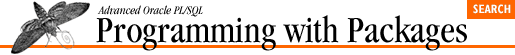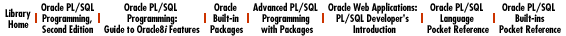home | O'Reilly's CD bookshelfs | FreeBSD | Linux | Cisco | Cisco Exam

### 14.4 Using PLVtmr in Scripts

In most situations, you will not place calls to PLVtmr inside your production code. Instead, you will extract specific elements of your application which you wish to focus on and understand their performance implications. You will usually write a SQL*Plus script that executes your code one or more times. If you do place the code within a loop, you should use the set_factor procedure to let PLVtmr know that it is timing multiple iterations of the code.

The following anonymous block, for example, calculates how long it takes to calculate totals. It also computes an average execution time over the specified number of iterations (passed in as a SQL*Plus argument) by calling the set_factor procedure:

``` BEGIN
PLVtmr.set_factor (&1);
PLVtmr.capture;
FOR rep IN 1 .. &1
LOOP
calc_totals;
END LOOP;
PLVtmr.show_elapsed ('calc_totals');
END;
/```

The PLVgen package will generate a loop like the one you see above. In fact, that script was generated with the following call in SQL*Plus:

`SQL> exec PLVgen.timer ('calc_totals');`

### 14.4.1 Comparing Performance of Different Implementations

Another common operation with PLVtmr is to compare two or more implementations of the same business rule or function. One example of this approach is shown below. In this script, I see which of my implementations of an "is number" function is most efficient. The first version is based on the TO_NUMBER builtin, while the second uses the LTRIM function.

```SET SERVEROUTPUT ON
SET VERIFY OFF
DECLARE
stg VARCHAR2(66) := '&2';
bool BOOLEAN;
BEGIN
PLVtmr.capture;
FOR i IN 1 .. &1
LOOP
bool := isnum.tonumber (stg);
IF i = 1 THEN do.pl(bool); END IF;
END LOOP;
PLVtmr.show_elapsed ('tonumber');

PLVtmr.capture;
FOR i IN 1 .. &1
LOOP
bool := isnum.trim (stg);
IF i = 1 THEN do.pl(bool); END IF;
END LOOP;
PLVtmr.show_elapsed ('trim');
END;
/```

### 14.4.2 Calculating Overhead of an Action

You can also use PLVtmr to calculate the overhead associated with a given operation. One example of this approach is shown by the func procedure of PLVtmr:

```PROCEDURE func
IS
myval NUMBER;
baseval NUMBER;
BEGIN
PLVtmr.capture;
FOR rep IN 1 .. v_repeats
LOOP
myval := 0;
END LOOP;
baseval := elapsed;
PLVtmr.capture;
FOR rep IN 1 .. v_repeats
LOOP
myval := numval;
END LOOP;
END;```

In this procedure, I compute the overhead associated with calling a function (versus making a direct assignment). I execute two different loops the number of times specified by v_repeats (which is set by the set_repeats procedure). In the first loop I obtain a baseline in which an assignment is executed. Rather than display that value, I simply assign to a local variable, baseval . I then execute a loop in which the function is called in place of the assignment. When this loop is completed, I display the elapsed time, passing the baseval variable as the amount by which the total elapsed time should be adjusted.

Here is an example of an execution of the func procedure three times, each based on 10,000 iterations. It shows that you can expect to incur upwards of 1/2 of one-thousandth of a second to make a function call (this was on a Pentium 90 Mhz laptop).

```SQL> exec PLVtmr.set_repeats(10000);
SQL> exec PLVtmr.func
Function Overhead Elapsed: 5.33 seconds. Factored: .00053 seconds.
SQL> exec PLVtmr.func
Function Overhead Elapsed: 4.73 seconds. Factored: .00047 seconds.
SQL> exec PLVtmr.func
Function Overhead Elapsed: 4.56 seconds. Factored: .00046 seconds.```

In fact, PLVtmr offers a number of programs to perform these kinds of comparisons: calibrate , currsucc , currfail , and, of course, func . In all cases, when you use PLVtmr to analyze performance, you should execute your test multiple times to make sure that your results stabilize around a consistent answer.14.3 Retrieving and Displaying the Elapsed Time15. PLVvu: Viewing Source Code and Compile Errors